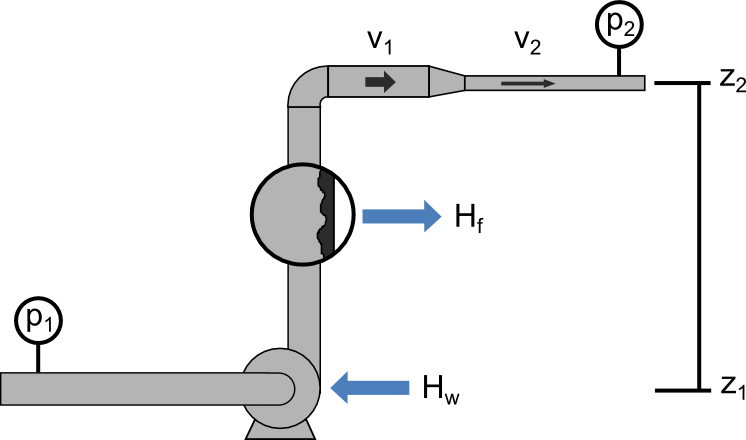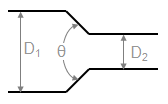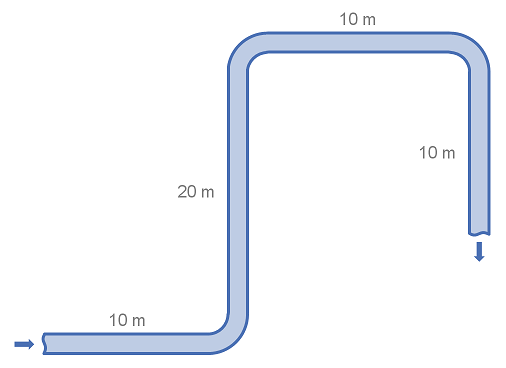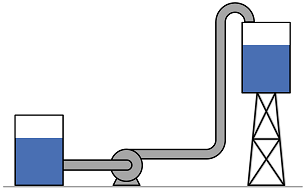f
Neutrium

Bernoulli's EquationBernoulli’s Principle is an important observation in fluid dynamics which states that for an inviscid flow, an increase in the velocity of the fluid results in a simultaneous decrease in pressure or a decrease in the fluid’s potential energy. This principle is often represented mathematically in the many forms of Bernoulli’s equation. This article presents some useful forms of Bernoulli’s Equations and their simplifying assumptions.

Pressure Loss from Fittings - Expansion and Reduction in Pipe SizeThis article provides methods to calculate the K-value (Resistance Coefficient) for determining the pressure loss cause by changes in the area of a fluid flow path. These types of pressure drops are highly dependent on the geometry and are not usually covered in simple pressure loss estimation schemes (such as a single k-value, equivalent length etc.)

Pressure Loss from Fittings - 3K MethodFittings such as elbows, tees, valves and reducers represent a significant component of the pressure loss in most pipe systems. This article details the calculation of pressure losses through pipe fittings and some minor equipment using the 3K method.

Pressure Loss from Fittings - 2K Method

Fittings such as elbows, tees, valves and reducers represent a significant component of the pressure loss in most pipe systems. This article details the calculation of pressure losses through pipe fittings and some minor equipment using the 2K method.

Pressure Loss from Fittings - Equivalent Length MethodFittings such as elbows, tees and valves represent a significant component of the pressure loss in most pipe systems. This article details the calculation of pressure losses through pipe fittings and some minor equipment using the equivalent length method. The strength of the equivalent length method is that it is very simple to calculate. The weakness of the equivalent length method is that it is not as accurate as other methods unless very detailed tabulated data is available.This article presents the method to convert between pressure and head for several common unit sets. Head relates the pressure of a fluid to the height of a column of that fluid which would produce an equivalent static pressure at its base. It is particularly useful for the specification of pumps as it provides a measure of pressure as it is independent of fluid density.

Pump Affinity LawsThe pump affinity laws allow the prediction of centrifugal pump performance given changes to the speed of the pump or the diameter of impeller. This article presents the pump affinity laws for reference.

Pump Cavitation and NPSH

Cavitation is the formation and subsequent collapse of vapour bubbles in a flowing liquid and is often responsible for significant damage flow equipment such as pumps. This article will provide an overview of cavitation and provide insight into the identification and prevention of cavitation conditions through the calculation of Net Positive Suction Head (NPSH).